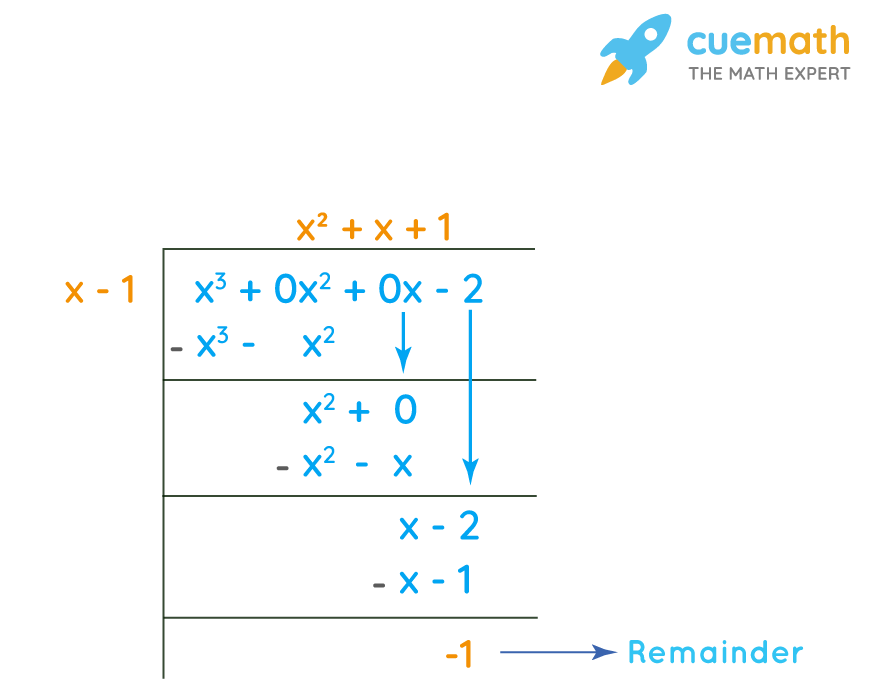# Find the remainder when (x^3 - 2) is divided by (x - 1).

## Question: Find the remainder when (x3 - 2) is divided by (x - 1).

The dividend here is (x3 - 2) and (x - 1) is the divisor.

## Answer: The remainder when (x3 - 2) is divided by (x - 1) is -1

Let's explore the long division of polynomials.

## Explanation:

The long division of polynomial (x3 - 2) divided by (x –-1) is given by:The remainder is -1, that means (x - 1) is not a factor of (x3 - 2).